National Institute of Standards and Technology
 Computational Chemistry Comparison and Benchmark DataBase Release 22 May 2022 NIST Standard Reference Database 101
 I Introduction II Experimental data III Calculated data IV Data comparisons V Cost comparisons VI Input and output files VII Tutorials and Units VIII Links to other sites IX Feedback X Older CCCBDB versions XII Geometries XIII Vibrations XIV Reaction data XV Entropy data XVI Bibliographic data XVII Ion data XVIII Bad calculations XIX Index of properties XX H-bond dimers XXI Oddities

NIST policy on privacy, security, and accessibility.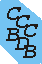A. Comparisons of Enthalpies 1. User specified reaction at 0K. a. User specified reaction at 298K. 2. Atomization enthalpies. a. Atomization enthalpies at 298K. 3. Methods which provide Enthalpy of formation directly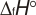a. Compare two semi-empirical methods. b. Compare semi-empirical and group additivity methods. 4. Relative enthaplies of isomers a. Compare sets of isomers. b. Compare sets of tautomers. 5. Barriers to internal rotation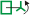B. Comparison of Entropies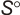1. Entropies for a given molecule2. Reaction Entropies at 298K 3. Heat Capacities (Cp) at 298K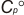4. Entropies for a selection of molecules5. Integrated heat capacities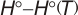C. Comparison of Vibrations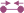1. Vibrational frequencies for a given molecule. 2. One particular vibrational frequency for a given molecule. 3. Calculate a vibrational scaling factor for a given set of molecules. 4. Notes on vibrational scaling factors 5. Vibrational zero point energies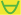6. Vibrational intensities for a given mode 7. Poorly predicted vibrational frequencies 8. Anharmonic vibrational frequencies for a given molecule D. Comparison of Geometries 1. Bonds, angles, or dihedrals for a given molecule.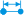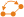2. Rotational constants for a given molecule.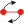3. Point Groups a. list molecules whose point groups differ from experiment 4. Bond or angle type for several molecules. 5. Products of Moments of Inertia.6. Bad calculated geometries.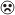7. Bad calculated bond lengths8. Average bond length differences by model chemistryE. Electrostatic Properties Comparisons 1. Electric dipoles.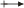2. Koopmans' theorem ionization energy predictions for a single species.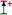3. Koopmans' theorem ionization energy predictions across a selection of species.4. Polarizabilities.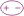5. Quadrupole moments.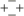6. Ionization Energy.7. Electron Affinity.8. Proton Affinity.